# PSAT Math : How to find the angle of two lines

## Example Questions

### Example Question #1 : Lines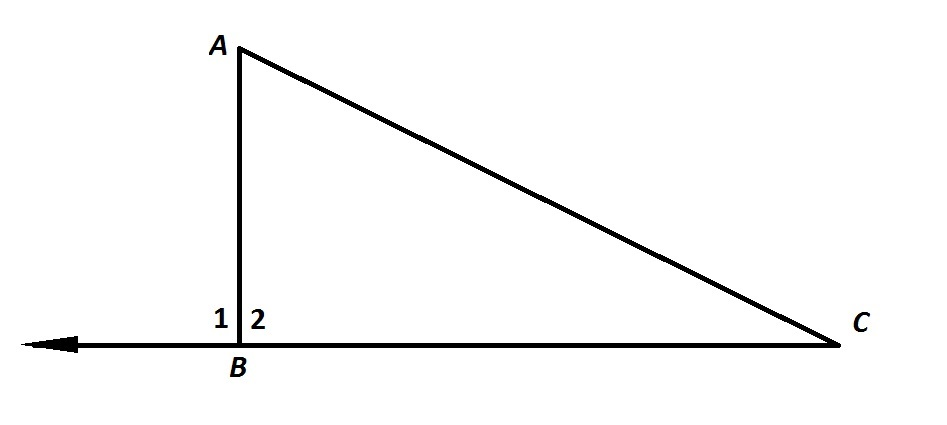Refer to the above figure. You are given that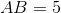and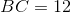. Which of the following statements would be sufficient to prove that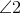is a right angle, given what is already known?

I)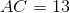II)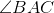and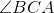are both acute

III)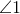is a right angle

I and II only

None of these

II and III only

I and III only

I, II, and III

I and III only

Explanation:

If, then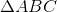has short sides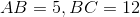and long side. Since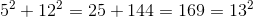,

then, by the converse of the Pythagorean Theorem,is a right triangle with right angle. Statement I is sufficient.

Ifandare both acute,we know nothing about; every triangle has at least two acute angles regardless of type. Statement II tells us nothing.andform a linear pair and are therefore supplementary. If one is a right angle, so is the other. Therefore, ifis a right angle, so is. Statement III is sufficient.

The correct response is Statement I and III only.

### Example Question #1 : How To Find The Angle Of Two Lines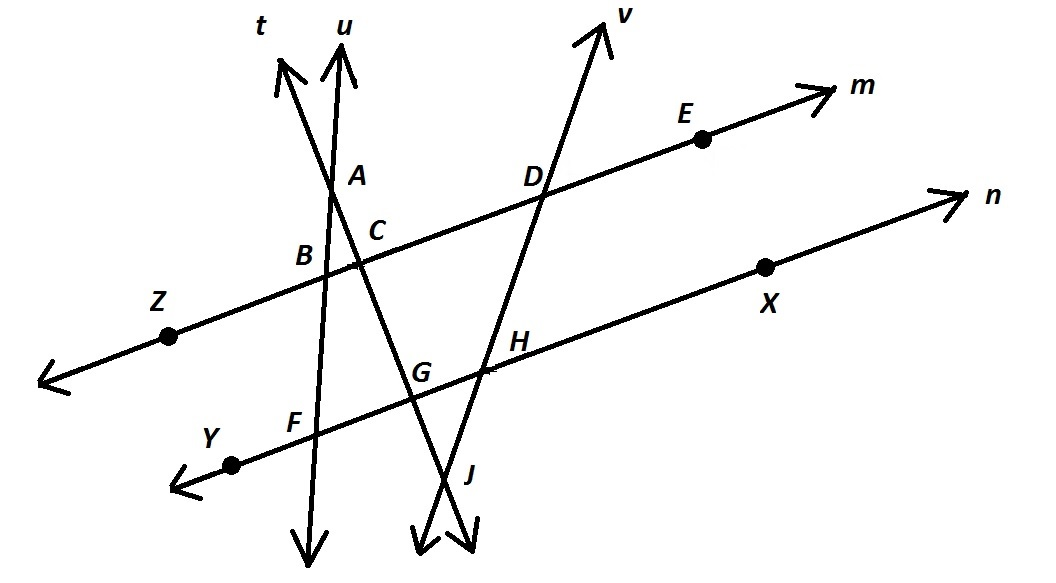Note: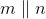Refer to the above diagram.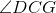and which other angle form a pair of corresponding angles?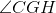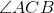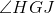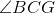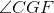Explanation:

Two angles formed by a transversal line crossing two other lines are corresponding angles if, relative to the points of intersection, they are in the same position.is formed by the intersection of transversaland; the angle in the same relative position whereintersectsis.

### Example Question #3 : LinesRefer to the above diagram.and which other angle form a pair of alternate interior angles?Explanation:

Two angles formed by a transversal line crossing two other lines are alternate interior angles if:

I) Both angles have their interiors between the lines crossed

II) The angles have their interiors on the opposite sides of the transversal.

Of the given choices, onlyfits the description; the interior of each is betweenand, and the interiors are on the opposite sides of.

### Example Question #4 : LinesRefer to the above diagram.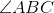and which other angle form a pair of vertical angles?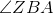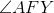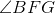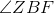Two angles are vertical angles if they share a vertex, anf if their union is a pair of intersecting lines. Of the five choices, onlyfits both descriptions with.